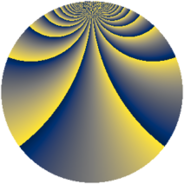# Properties

 Label 2880.2.ffLevel $2880$ Weight $2$ Character orbit 2880.ff Rep. character $\chi_{2880}(11,\cdot)$ Character field $\Q(\zeta_{48})$ Dimension $6144$ Sturm bound $1152$

# Learn more about

## Defining parameters

 Level: $$N$$ $$=$$ $$2880 = 2^{6} \cdot 3^{2} \cdot 5$$ Weight: $$k$$ $$=$$ $$2$$ Character orbit: $$[\chi]$$ $$=$$ 2880.ff (of order $$48$$ and degree $$16$$) Character conductor: $$\operatorname{cond}(\chi)$$ $$=$$ $$576$$ Character field: $$\Q(\zeta_{48})$$ Sturm bound: $$1152$$

## Dimensions

The following table gives the dimensions of various subspaces of $$M_{2}(2880, [\chi])$$.

Total New Old
Modular forms 9280 6144 3136
Cusp forms 9152 6144 3008
Eisenstein series 128 0 128

## Trace form

 $$6144q + O(q^{10})$$ $$6144q + 160q^{24} + 160q^{42} - 144q^{58} + 48q^{76} + 48q^{78} + 112q^{84} + 144q^{90} + 272q^{96} + O(q^{100})$$

## Decomposition of $$S_{2}^{\mathrm{new}}(2880, [\chi])$$ into newform subspaces

The newforms in this space have not yet been added to the LMFDB.

## Decomposition of $$S_{2}^{\mathrm{old}}(2880, [\chi])$$ into lower level spaces

$$S_{2}^{\mathrm{old}}(2880, [\chi]) \cong$$ $$S_{2}^{\mathrm{new}}(576, [\chi])$$$$^{\oplus 2}$$